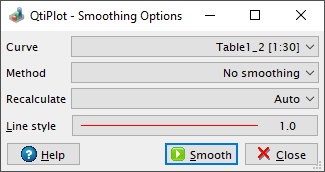# Smoothing Options

This dialog can be used in order to perform a smoothing of the input data set selected in the Curve list box, using one of the following methods:

Figure 5-126. Comparison of the curve smoothing methods available in QtiPlot applied to a simulated sine curve with added white noise.1) Savitski-Golay:

The Analysis -> Smooth -> Savitsky-Golay... command opens this dialog with the Savitzky-Golay method selected. The formula used to smooth the curve defined by the points yi=f(xi) is in this case:The fi values are computed by fitting the data points to a polynomial. They depend on the number of points used for the smoothing of the curve and the order of the polynomial. Compared to the moving window average method, the advantage of this smoothing method is that the values of extrema are not truncated. The dialog allows specification of the order of the polynomial and the number of data points used for the polynomial fit before and after each point.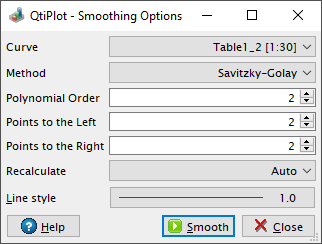2) Moving Window Average:

The Analysis -> Smooth -> Moving Window Average... command opens this dialog with the moving window average method already selected. The formula used to smooth the curve defined by the points yi=f(xi) is in this case: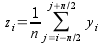The greater the number of averaged points, n, the smoother the resulting curve zi=f(xi) will be. The dialog allows specification of the value of n via the Points input box. Please note that the algorithm implemented in QtiPlot always uses an odd number of averaged points, even if an even number is specified by the user.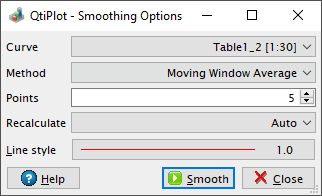3) Lowess:

The Analysis -> Smooth -> Lowess... command opens this dialog with the Lowess (aka Loess) method already selected. This method provides a robust locally weighted regression and is well suited to smooth data for which no formal model exists.

The parameter f is the fraction of points which define the local neighborhood. A value of 0.2 uses 20% of the curve total points as neighbors for each data point (+/- 10%). Values of f closer to 1 yield smoother curves. The Iterations parameter specifies the number of times the algorithm runs over the entire data set, each time refining the local weights. In most cases, two iterations are sufficient.4) FFT Filter:

The Analysis -> Smooth -> FFT Filter... command opens this dialog with the FFT Filter method already selected. This method performs a smoothing of the selected curve using a Low Pass technique: the number of Points specified is used to calculate a cut-off frequency.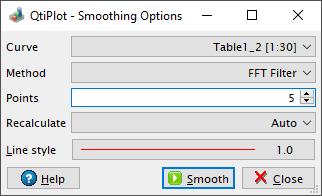For all methods, this dialog allows specification of the line style used to draw the smoothed curved. A new hidden table is created in order to store the output data points xi, zi of the smoothed curve. If the selected method is No smoothing, the output of the smoothing filter is a curve identical with the input data curve.